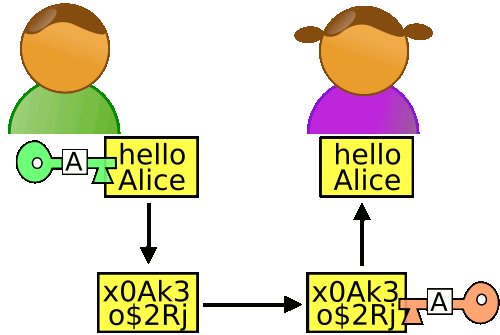# Rsa

Posted by SunQH Blog on October 11, 2019

# RSA

RSA 是一种广泛使用的非对称加密算法。非对称加密包含三个部分: 公钥和私钥的生成，使用公钥加密数据，使用私钥解密数据。

• 乙方生成两把密钥（公钥和私钥）。公钥是公开的，任何人都可以获得，私钥则是保密的。
• 甲方获取乙方的公钥，然后用它对信息加密。
• 乙方得到加密后的信息，用私钥解密。### 密钥的生成

Step 1: 随机选择两个不相等的质数p和q。

Step 2: 计算p和q的乘积n $$n = 61×53 = 3233$$ Step 3: 计算n的欧拉函数φ(n)。

$$φ(n) = (p-1)(q-1) = 60 \times 52 = 3120$$ Step 4: 随机选择一个整数e，条件是1< e < φ(n)，且e与φ(n) 互质

Step 5: 计算e对于φ(n)的模反元素d。

### 解密